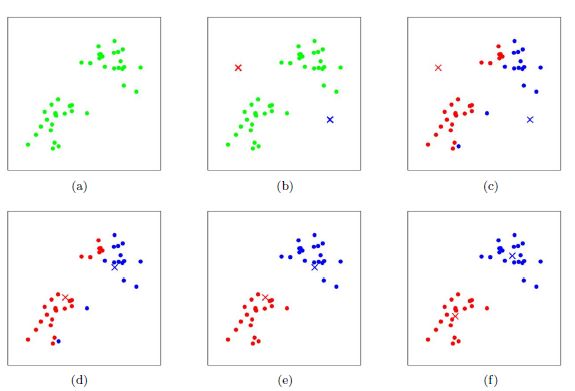K-means在Python中的实现

Stardustsky   2017-08-25 17:01   1145 人阅读  0 条评论

K-means算法简介

K-means是机器学习中一个比较常用的算法，属于无监督学习算法，其常被用于数据的聚类，只需为它指定簇的数量即可自动将数据聚合到多类中，相同簇中的数据相似度较高，不同簇中数据相似度较低。

K-menas的优缺点：

• 原理简单
• 速度快
• 对大数据集有比较好的伸缩性

• 需要指定聚类 数量K
• 对异常值敏感
• 对初始值敏感

K-means的聚类过程

1. 适当选择c个类的初始中心；
2. 在第k次迭代中，对任意一个样本，求其到c个中心的距离，将该样本归到距离最短的中心所在的类；
3. 利用均值等方法更新该类的中心值；
4. 对于所有的c个聚类中心，如果利用（2）（3）的迭代法更新后，值保持不变，则迭代结束，否则继续迭代。K-means 实例展示

python中km的一些参数：

``````sklearn.cluster.KMeans(
n_clusters=8,
init='k-means++',
n_init=10,
max_iter=300,
tol=0.0001,
precompute_distances='auto',
verbose=0,
random_state=None,
copy_x=True,
n_jobs=1,
algorithm='auto'
)
n_clusters: 簇的个数，即你想聚成几类
init: 初始簇中心的获取方法
n_init: 获取初始簇中心的更迭次数，为了弥补初始质心的影响，算法默认会初始10个质心，实现算法，然后返回最好的结果。
max_iter: 最大迭代次数（因为kmeans算法的实现需要迭代）
tol: 容忍度，即kmeans运行准则收敛的条件
precompute_distances:是否需要提前计算距离，这个参数会在空间和时间之间做权衡，如果是True 会把整个距离矩阵都放到内存中，auto 会默认在数据样本大于featurs*samples 的数量大于12e6 的时候False,False 时核心实现的方法是利用Cpython 来实现的
verbose: 冗长模式（不太懂是啥意思，反正一般不去改默认值）
random_state: 随机生成簇中心的状态条件。
copy_x: 对是否修改数据的一个标记，如果True，即复制了就不会修改数据。bool 在scikit-learn 很多接口中都会有这个参数的，就是是否对输入数据继续copy 操作，以便不修改用户的输入数据。这个要理解Python 的内存机制才会比较清楚。
n_jobs: 并行设置
algorithm: kmeans的实现算法，有：’auto’, ‘full’, ‘elkan’, 其中 ‘full’表示用EM方式实现

``````from sklearn.cluster import KMeans
from sklearn.externals import joblib
from sklearn import cluster
import numpy as np

# 生成10*3的矩阵
data = np.random.rand(10,3)
print data
# 聚类为4类
estimator=KMeans(n_clusters=4)
# fit_predict表示拟合+预测，也可以分开写
res=estimator.fit_predict(data)
# 预测类别标签结果
lable_pred=estimator.labels_
# 各个类别的聚类中心值
centroids=estimator.cluster_centers_
# 聚类中心均值向量的总和
inertia=estimator.inertia_

print lable_pred
print centroids
print inertia

[0 2 1 0 2 2 0 3 2 0]

[[ 0.3028348   0.25183096  0.62493622]
[ 0.88481287  0.70891813  0.79463764]
[ 0.66821961  0.54817207  0.30197415]
[ 0.11629904  0.85684903  0.7088385 ]]

0.570794546829``````

``````from sklearn.cluster import KMeans
from sklearn.externals import joblib
from sklearn import cluster
import numpy as np
import matplotlib.pyplot as plt

data = np.random.rand(100,2)
estimator=KMeans(n_clusters=3)
res=estimator.fit_predict(data)
lable_pred=estimator.labels_
centroids=estimator.cluster_centers_
inertia=estimator.inertia_
#print res
print lable_pred
print centroids
print inertia

for i in range(len(data)):
if int(lable_pred[i])==0:
plt.scatter(data[i],data[i],color='red')
if int(lable_pred[i])==1:
plt.scatter(data[i],data[i],color='black')
if int(lable_pred[i])==2:
plt.scatter(data[i],data[i],color='blue')
plt.show()``````10000条 4s 50维
100000条 30s 50维
1000000条 4'13s 50维

``````from sklearn.externals import joblib
joblib.dump(km,"model/km_model.m")``````

相关文章